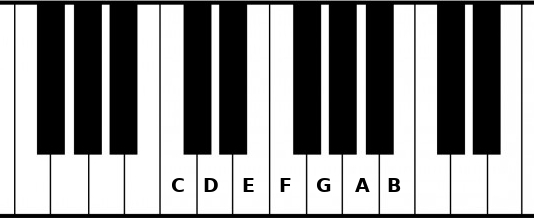This is the second part of the music theory in F# series:

## Theory

There are 12 notes in an octave, but if I play random notes, the sound of the melody will be closer to a piano falling down the stairs than a Beethoven sonata. Fortunately, scales will help us.

The most famous scale is the major scale. A scale has a starting note named root and other notes after it. In example the C major scale is:

C, D, E, F, G, A, B

A scale is defined by the intervals between each notes. To create a major scale, choose a root note and add these intervals:

Whole tone, semitone, whole tone, whole tone, whole tone, semitone

For instance, to create a C major:

``````Root:    C
Second:  D = C + 2 semitones
Third:   E = D + 2 semitones
Fourth:  F = E + 1 semitone
Fifth:   G = F + 2 semitones
Sixth:   A = G + 2 semitones
Seventh: B = A + 2 semitones
Octave:  C = B + 1 semitone
``````Each note of the scale is named after its position (root, second, third…). You can now create any major scale by applying these intervals. Here is another example, E major:

``````Root:    E
Second:  F# = E  + 2 semitones
Third:   G# = F# + 2 semitones
Fourth:  A  = G# + 1 semitone
Fifth:   B  = A  + 2 semitones
Sixth:   C# = B  + 2 semitones
Seventh: D# = C# + 2 semitones
Octave:  E  = D# + 1 semitone
``````

And so on…

## Code

We can start by designing the `MajorScale` type and its module.

``````type MajorScale = MajorScale of Note

module MajorScale =
let private intervals = [ 2; 2; 1; 2; 2; 2; 1 ]
``````

Remember the union type for the `Note` type? It can be seen as an enum, but unions are more than that because each case can have its own data:

``````type Shape =
| Point
| Square of int
| Rectangle of int * int // int * int is the type for a tuple of int and int

// All these values are Shape
let point = Point
let square = Square 3
let rect = Rectangle (4, 5) // (4, 5) is a value for a tuple of int and int
``````

Well, `MajorScale` is a single union. It’s a trick to wrap a type into another one with a clear intention. More explanations in this article.

Now we want a `notes` function that takes a `MajorScale` and return the list of its `Notes`. In the theory part, we saw that the algorithm to find all the notes behave like this:

``````Return the root note
foreach interval in intervals
return the previous note + interval
``````

This function already exists, it is called `List.scan`. It takes 3 parameters:

• The list to iterate (`intervals`)
• The starting point (`root note`)
• The function to compute the new value from the current item and the previous value (`previous note` + `interval`)
``````module MajorScale =
let private intervals = [ 2; 2; 1; 2; 2; 2; 1 ]

let notes (MajorScale root) =
let addSemitones note interval = // not implemented yet

intervals

// expected behavior
let cMajorNotes = MajorScale C |> notes //  [ C; D; E; F; G; A; B ]
``````

The function `notes` takes a `MajorScale` as parameter and destructures it. By doing this, we can easily access to `root` of the scale.

Then `addSemitones` is a function that takes a note and an interval and return the new note:

``````// expected behavior
let dSharp = addSemitones D 1 // DSharp
``````

With this function implemented, `notes` called on a `MajorScale C` will return:

``````[ C; D; E; F; G; A; B; C ]
``````

`C` appears two times because the last interval `1` is the interval from the seventh to the octave. We don’t need it to get all the notes, so we can remove the last interval:

``````module MajorScale =
let private intervals = [ 2; 2; 1; 2; 2; 2; 1 ]

let notes (MajorScale root) =
let addSemitones note interval = // not implemented yet

intervals
|> List.take (intervals.Length - 1)
``````

Let’s implement `addSemitones`:

``````module Note =
// find the index where the item of the list equals note
let index =
all
|> List.findIndex ((=) note)

// add the semitones to the index
// and get only the rest of the division
let newIndex = (index + semitones) % all.Length

all[newIndex]
``````

and call this function in the `notes` function:

``````module MajorScale =
let notes (MajorScale root) =
intervals
|> List.take (intervals.Length - 1)
``````

At the end of the script, we can print the notes of the scale:

``````// Add this helper on top of the file, just after the open statements
module String =
let joinWith (separator: string) (strings: string list) =
String.Join(separator, strings)

MajorScale randomNote
|> MajorScale.notes
|> List.map Note.toString
|> String.joinWith ", "
|> printfn "Notes of the major scale: %s"

// With randomNote = C, it prints:
// Notes of the major scale: C, D, E, F, G, A, B
``````

Yay, now we can play random notes in this scale and it sound good!

## Conclusion

That’s all for this step. You can check out the full code here.

Next step: Chords of the Major scale